Test: Syllogism- 3

# Test: Syllogism- 3

Test Description

## 30 Questions MCQ Test CSAT Preparation for UPSC CSE | Test: Syllogism- 3

Test: Syllogism- 3 for SSC CGL 2023 is part of CSAT Preparation for UPSC CSE preparation. The Test: Syllogism- 3 questions and answers have been prepared according to the SSC CGL exam syllabus.The Test: Syllogism- 3 MCQs are made for SSC CGL 2023 Exam. Find important definitions, questions, notes, meanings, examples, exercises, MCQs and online tests for Test: Syllogism- 3 below.
Solutions of Test: Syllogism- 3 questions in English are available as part of our CSAT Preparation for UPSC CSE for SSC CGL & Test: Syllogism- 3 solutions in Hindi for CSAT Preparation for UPSC CSE course. Download more important topics, notes, lectures and mock test series for SSC CGL Exam by signing up for free. Attempt Test: Syllogism- 3 | 30 questions in 30 minutes | Mock test for SSC CGL preparation | Free important questions MCQ to study CSAT Preparation for UPSC CSE for SSC CGL Exam | Download free PDF with solutions
 1 Crore+ students have signed up on EduRev. Have you?
Test: Syllogism- 3 - Question 1

### Directions to Solve In each of the following questions two statements are given and these statements are followed by two conclusions numbered (1) and (2). You have to take the given two statements to be true even if they seem to be at variance from commonly known facts. Read the conclusions and then decide which of the given conclusions logically follows from the two given statements, disregarding commonly known facts. Give answer: (A) If only (1) conclusion follows (B) If sonly (2) conclusion follows (C) If either (1) or (2) follows (D) If neither (1) nor (2) follows and (E) If both (1) and (2) follow. Question - Statements: Some actors are singers. All the singers are dancers. Conclusions: Some actors are dancers. No singer is actor.

Detailed Solution for Test: Syllogism- 3 - Question 1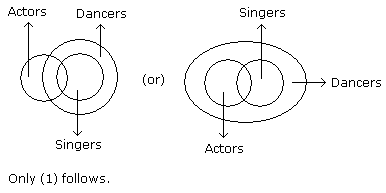Test: Syllogism- 3 - Question 2

### Directions to Solve In each of the following questions two statements are given and these statements are followed by two conclusions numbered (1) and (2). You have to take the given two statements to be true even if they seem to be at variance from commonly known facts. Read the conclusions and then decide which of the given conclusions logically follows from the two given statements, disregarding commonly known facts. Give answer: (A) If only (1) conclusion follows (B) If sonly (2) conclusion follows (C) If either (1) or (2) follows (D) If neither (1) nor (2) follows and (E) If both (1) and (2) follow. Question - Statements: All the harmoniums are instruments. All the instruments are flutes. Conclusions: All the flutes are instruments. All the harmoniums are flutes.

Detailed Solution for Test: Syllogism- 3 - Question 2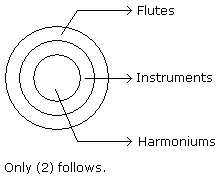Test: Syllogism- 3 - Question 3

### Directions to Solve In each of the following questions two statements are given and these statements are followed by two conclusions numbered (1) and (2). You have to take the given two statements to be true even if they seem to be at variance from commonly known facts. Read the conclusions and then decide which of the given conclusions logically follows from the two given statements, disregarding commonly known facts. Give answer: (A) If only (1) conclusion follows (B) If sonly (2) conclusion follows (C) If either (1) or (2) follows (D) If neither (1) nor (2) follows and (E) If both (1) and (2) follow. Question - Statements: Some mangoes are yellow. Some tixo are mangoes. Conclusions: Some mangoes are green. Tixo is a yellow.

Detailed Solution for Test: Syllogism- 3 - Question 3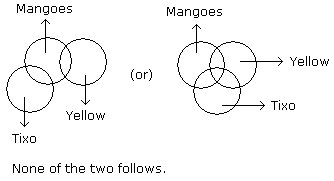Test: Syllogism- 3 - Question 4

Directions to Solve

In each of the following questions two statements are given and these statements are followed by two conclusions numbered (1) and (2). You have to take the given two statements to be true even if they seem to be at variance from commonly known facts. Read the conclusions and then decide which of the given conclusions logically follows from the two given statements, disregarding commonly known facts.

• (A) If only (1) conclusion follows
• (B) If sonly (2) conclusion follows
• (C) If either (1) or (2) follows
• (D) If neither (1) nor (2) follows and
• (E) If both (1) and (2) follow.

Question -

Statements: Some papers are pens. All the pencils are pens.

Conclusions:

1. Some pens are pencils.
2. Some pens are papers.
Detailed Solution for Test: Syllogism- 3 - Question 4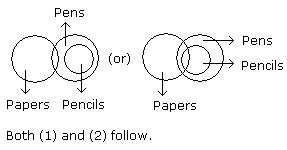Test: Syllogism- 3 - Question 5

Directions to Solve

In each of the following questions two statements are given and these statements are followed by two conclusions numbered (1) and (2). You have to take the given two statements to be true even if they seem to be at variance from commonly known facts. Read the conclusions and then decide which of the given conclusions logically follows from the two given statements, disregarding commonly known facts.

• (A) If only (1) conclusion follows
• (B) If sonly (2) conclusion follows
• (C) If either (1) or (2) follows
• (D) If neither (1) nor (2) follows and
• (E) If both (1) and (2) follow.

Question -

Statements: Some ants are parrots. All the parrots are apples.

Conclusions:

1. All the apples are parrots.
2. Some ants are apples.
Detailed Solution for Test: Syllogism- 3 - Question 5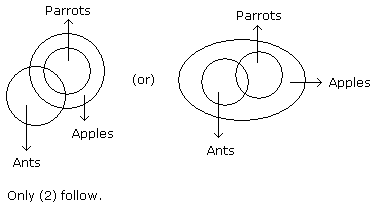Test: Syllogism- 3 - Question 6

Directions to Solve

In each of the following questions two statements are given and these statements are followed by two conclusions numbered (1) and (2). You have to take the given two statements to be true even if they seem to be at variance from commonly known facts. Read the conclusions and then decide which of the given conclusions logically follows from the two given statements, disregarding commonly known facts.

• (A) If only (1) conclusion follows
• (B) If sonly (2) conclusion follows
• (C) If either (1) or (2) follows
• (D) If neither (1) nor (2) follows and
• (E) If both (1) and (2) follow.

Question -

Statements: All the actors are girls. All the girls are beautiful.

Conclusions:

1. All the actors are beautiful.
2. Some girls are actors.
Detailed Solution for Test: Syllogism- 3 - Question 6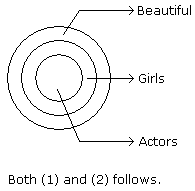Test: Syllogism- 3 - Question 7

Directions to Solve

In each of the following questions two statements are given and these statements are followed by two conclusions numbered (1) and (2). You have to take the given two statements to be true even if they seem to be at variance from commonly known facts. Read the conclusions and then decide which of the given conclusions logically follows from the two given statements, disregarding commonly known facts.

• (A) If only (1) conclusion follows
• (B) If sonly (2) conclusion follows
• (C) If either (1) or (2) follows
• (D) If neither (1) nor (2) follows and
• (E) If both (1) and (2) follow.

Question -

Statements: All the windows are doors. No door is a wall.

Conclusions:

1. Some windows are walls.
2. No wall is a door.
Detailed Solution for Test: Syllogism- 3 - Question 7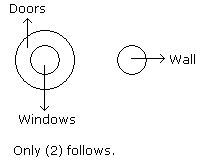Test: Syllogism- 3 - Question 8

Directions to Solve

In each of the following questions two statements are given and these statements are followed by two conclusions numbered (1) and (2). You have to take the given two statements to be true even if they seem to be at variance from commonly known facts. Read the conclusions and then decide which of the given conclusions logically follows from the two given statements, disregarding commonly known facts.

• (A) If only (1) conclusion follows
• (B) If sonly (2) conclusion follows
• (C) If either (1) or (2) follows
• (D) If neither (1) nor (2) follows and
• (E) If both (1) and (2) follow.

Question -

Statements: All cups are books. All books are shirts.

Conclusions:

1. Some cups are not shirts.
2. Some shirts are cups.
Detailed Solution for Test: Syllogism- 3 - Question 8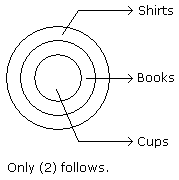Test: Syllogism- 3 - Question 9

Directions to Solve

In each of the following questions two statements are given and these statements are followed by two conclusions numbered (1) and (2). You have to take the given two statements to be true even if they seem to be at variance from commonly known facts. Read the conclusions and then decide which of the given conclusions logically follows from the two given statements, disregarding commonly known facts.

• (A) If only (1) conclusion follows
• (B) If sonly (2) conclusion follows
• (C) If either (1) or (2) follows
• (D) If neither (1) nor (2) follows and
• (E) If both (1) and (2) follow.

Question -

Statements: Some cows are crows. Some crows are elephants.

Conclusions:

1. Some cows are elephants.
2. All crows are elephants.
Detailed Solution for Test: Syllogism- 3 - Question 9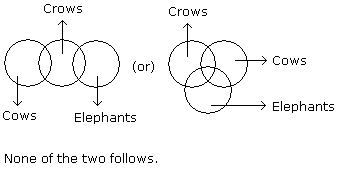Test: Syllogism- 3 - Question 10

Directions to Solve

In each of the following questions two statements are given and these statements are followed by two conclusions numbered (1) and (2). You have to take the given two statements to be true even if they seem to be at variance from commonly known facts. Read the conclusions and then decide which of the given conclusions logically follows from the two given statements, disregarding commonly known facts.

• (A) If only (1) conclusion follows
• (B) If sonly (2) conclusion follows
• (C) If either (1) or (2) follows
• (D) If neither (1) nor (2) follows and
• (E) If both (1) and (2) follow.

Question -

Statements: All the pencils are pens. All the pens are inks.

Conclusions:

1. All the pencils are inks.
2. Some inks are pencils.
Detailed Solution for Test: Syllogism- 3 - Question 10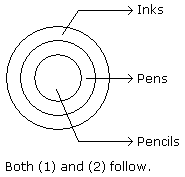Test: Syllogism- 3 - Question 11

Directions to Solve

In each of the following questions two statements are given and these statements are followed by two conclusions numbered (1) and (2). You have to take the given two statements to be true even if they seem to be at variance from commonly known facts. Read the conclusions and then decide which of the given conclusions logically follows from the two given statements, disregarding commonly known facts.

• (A) If only (1) conclusion follows
• (B) If sonly (2) conclusion follows
• (C) If either (1) or (2) follows
• (D) If neither (1) nor (2) follows and
• (E) If both (1) and (2) follow.

Question -

Statements: Some dogs are bats. Some bats are cats.

Conclusions:

1. Some dogs are cats.
2. Some cats are dogs.
Detailed Solution for Test: Syllogism- 3 - Question 11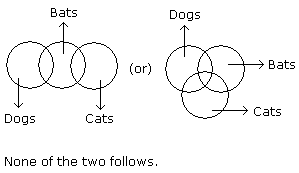Test: Syllogism- 3 - Question 12

Directions to Solve

In each of the following questions two statements are given and these statements are followed by two conclusions numbered (1) and (2). You have to take the given two statements to be true even if they seem to be at variance from commonly known facts. Read the conclusions and then decide which of the given conclusions logically follows from the two given statements, disregarding commonly known facts.

• (A) If only (1) conclusion follows
• (B) If sonly (2) conclusion follows
• (C) If either (1) or (2) follows
• (D) If neither (1) nor (2) follows and
• (E) If both (1) and (2) follow.

Question -

Statements: All the trucks are flies. Some scooters are flies.

Conclusions:

1. All the trucks are scooters.
2. Some scooters are trucks.
Detailed Solution for Test: Syllogism- 3 - Question 12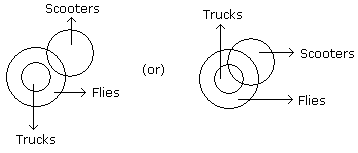Neither (1) nor (2) follows.

Test: Syllogism- 3 - Question 13

Directions to Solve

In each of the following questions two statements are given and these statements are followed by two conclusions numbered (1) and (2). You have to take the given two statements to be true even if they seem to be at variance from commonly known facts. Read the conclusions and then decide which of the given conclusions logically follows from the two given statements, disregarding commonly known facts.

• (A) If only (1) conclusion follows
• (B) If sonly (2) conclusion follows
• (C) If either (1) or (2) follows
• (D) If neither (1) nor (2) follows and
• (E) If both (1) and (2) follow.

Question -

Statements: All buildings are chalks. No chalk is toffee.

Conclusions:

1. No building is toffee
2. All chalks are buildings.
Detailed Solution for Test: Syllogism- 3 - Question 13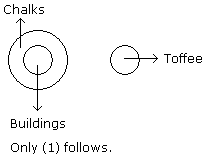Test: Syllogism- 3 - Question 14

Directions to Solve

In each of the following questions two statements are given and these statements are followed by two conclusions numbered (1) and (2). You have to take the given two statements to be true even if they seem to be at variance from commonly known facts. Read the conclusions and then decide which of the given conclusions logically follows from the two given statements, disregarding commonly known facts.

• (A) If only (1) conclusion follows
• (B) If sonly (2) conclusion follows
• (C) If either (1) or (2) follows
• (D) If neither (1) nor (2) follows and
• (E) If both (1) and (2) follow.

Question -

Statements: All cars are cats. All fans are cats.

Conclusions:

1. All cars are fans.
2. Some fans are cars.
Detailed Solution for Test: Syllogism- 3 - Question 14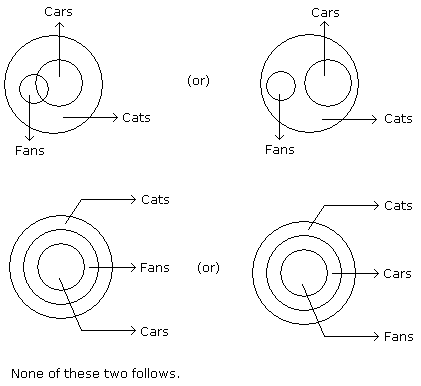Test: Syllogism- 3 - Question 15

Directions to Solve

In each of the following questions two statements are given. Which are followed by four conclusions (1), (2), (3) and (4). Choose the conclusions which logically follow from the given statements.

Question -

Statements: No door is dog. All the dogs are cats.

Conclusions:

1. No door is cat.
2. No cat is door.
3. Some cats are dogs.
4. All the cats are dogs.
Detailed Solution for Test: Syllogism- 3 - Question 15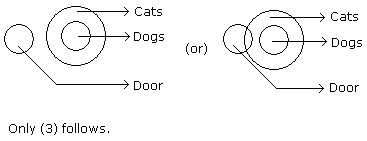Test: Syllogism- 3 - Question 16

Directions to Solve

In each of the following questions two statements are given. Which are followed by four conclusions (1), (2), (3) and (4). Choose the conclusions which logically follow from the given statements.

Question -

Statements: All green are blue. All blue are white.

Conclusions:

1. Some blue are green.
2. Some white are green.
3. Some green are not white.
4. All white are blue.
Detailed Solution for Test: Syllogism- 3 - Question 16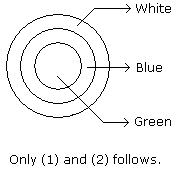Test: Syllogism- 3 - Question 17

Directions to Solve

In each of the following questions two statements are given. Which are followed by four conclusions (1), (2), (3) and (4). Choose the conclusions which logically follow from the given statements.

Question -

Statements: All men are vertebrates. Some mammals are vertebrates.

Conclusions:

1. All men are mammals.
2. All mammals are men.
3. Some vertebrates are mammals.
4. All vertebrates are men.
Detailed Solution for Test: Syllogism- 3 - Question 17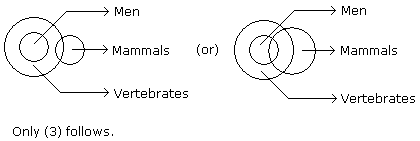Test: Syllogism- 3 - Question 18

Directions to Solve

In each of the following questions two statements are given. Which are followed by four conclusions (1), (2), (3) and (4). Choose the conclusions which logically follow from the given statements.

Question -

Statements: All the phones are scales. All the scales are calculators.

Conclusions:

1. All the calculators are scales.
2. All the phones are calculators
3. All the scales are phones.
4. Some calculators are phones.
Detailed Solution for Test: Syllogism- 3 - Question 18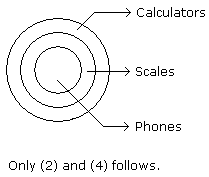Test: Syllogism- 3 - Question 19

Directions to Solve

In each of the following questions two statements are given. Which are followed by four conclusions (1), (2), (3) and (4). Choose the conclusions which logically follow from the given statements.

Question -

Statements: Some tables are T.V. Some T.V. are radios.

Conclusions:

3. All the radios are T.V.
4. All the T.V. are tables.
Detailed Solution for Test: Syllogism- 3 - Question 19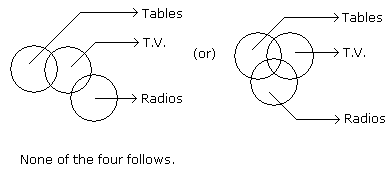Test: Syllogism- 3 - Question 20

Directions to Solve

In each of the following questions two statements are given. Which are followed by four conclusions (1), (2), (3) and (4). Choose the conclusions which logically follow from the given statements.

Question -

Statements: Some pens are books. Some books are pencils.

Conclusions:

1. Some pens are pencils.
2. Some pencils are pens.
3. All pencils are pens.
4. All books are pens.
Detailed Solution for Test: Syllogism- 3 - Question 20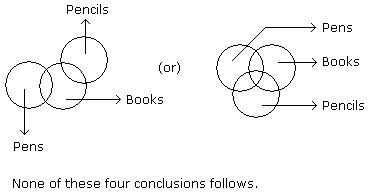Test: Syllogism- 3 - Question 21

Directions to Solve

In each of the following questions two statements are given. Which are followed by four conclusions (1), (2), (3) and (4). Choose the conclusions which logically follow from the given statements.

Question -

Statements: All the goats are tigers. All the tigers are lions.

Conclusions:

1. All the goats are lions.
2. All the lions are goats.
3. Some lions are goats.
4. Some tigers are goats.
Detailed Solution for Test: Syllogism- 3 - Question 21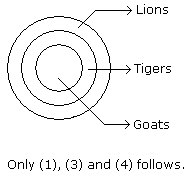Test: Syllogism- 3 - Question 22

Directions to Solve

In each of the following questions two statements are given. Which are followed by four conclusions (1), (2), (3) and (4). Choose the conclusions which logically follow from the given statements.

Question -

Statements: All the books are pencils. No pencil is eraser.

Conclusions:

1. All the pencils are books.
2. Some erasers are books.
3. No book is eraser.
4. Some books are erasers.
Detailed Solution for Test: Syllogism- 3 - Question 22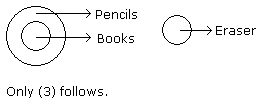Test: Syllogism- 3 - Question 23

Directions to Solve

In each of the following questions two statements are given. Which are followed by four conclusions (1), (2), (3) and (4). Choose the conclusions which logically follow from the given statements.

Question -

Statements: All the research scholars are psychologists. Some psychologists are scientists.

Conclusions:

1. All the research scholars are scientists.
2. Some research scholars are scientists.
3. Some scientists are psychologists.
4. Some psychologists are research scholars.
Detailed Solution for Test: Syllogism- 3 - Question 23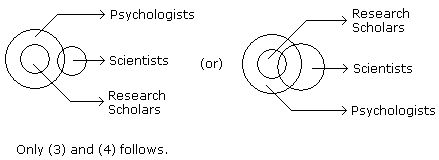Test: Syllogism- 3 - Question 24

Directions to Solve

In each of the following questions there are three statements. Which are followed by three or four conclusions. Choose the conclusions which logically follow from the given statements.

Question -

Statements: All the locks are keys. All the keys are bats. Some watches are bats.

Conclusions:

1. Some bats are locks.
2. Some watches are keys.
3. All the keys are locks.
Detailed Solution for Test: Syllogism- 3 - Question 24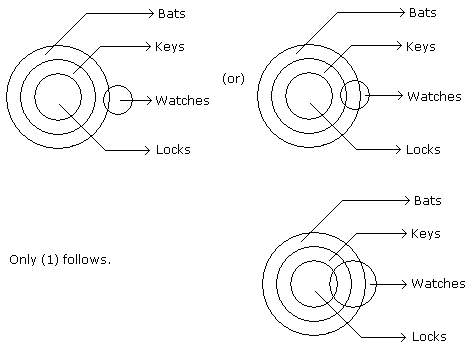Test: Syllogism- 3 - Question 25

Directions to Solve

In each of the following questions there are three statements. Which are followed by three or four conclusions. Choose the conclusions which logically follow from the given statements.

Question -

Statements: Some keys are staplers. Some staplers are stickers. All the stickers are pens.

Conclusions:

1. Some pens are staplers.
2. Some stickers are keys.
3. No sticker is key.
4. Some staplers are keys.
Detailed Solution for Test: Syllogism- 3 - Question 25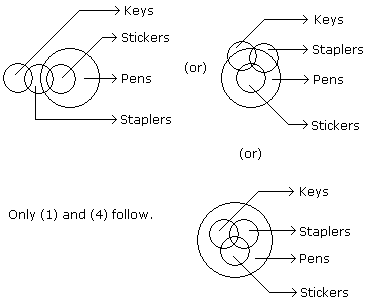Test: Syllogism- 3 - Question 26

Directions to Solve

In each of the following questions there are three statements. Which are followed by three or four conclusions. Choose the conclusions which logically follow from the given statements.

Question -

Statements: Some questions are answers. Some answers are writers. All the writers are poets.

Conclusions:

2. Some poets are questions.
3. All the questions are poets.
Detailed Solution for Test: Syllogism- 3 - Question 26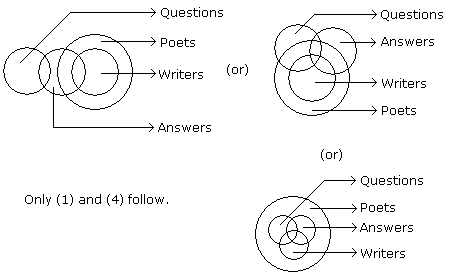Test: Syllogism- 3 - Question 27

Directions to Solve

In each of the following questions there are three statements. Which are followed by three or four conclusions. Choose the conclusions which logically follow from the given statements.

Question -

Statements: Some envelops are gums. Some gums are seals. Some seals are adhesives.

Conclusions:

1. Some envelopes are seals.
Detailed Solution for Test: Syllogism- 3 - Question 27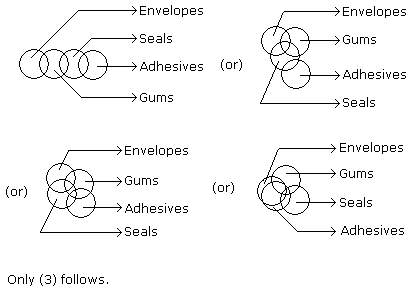Test: Syllogism- 3 - Question 28

Directions to Solve

In each of the following questions there are three statements. Which are followed by three or four conclusions. Choose the conclusions which logically follow from the given statements.

Question -

Statements: All the papers are books. All the bags are books. Some purses are bags.

Conclusions:

1. Some papers are bags.
2. Some books are papers.
3. Some books are purses.
Detailed Solution for Test: Syllogism- 3 - Question 28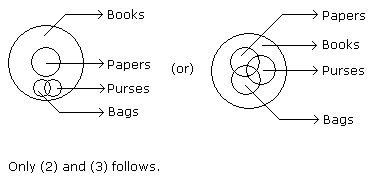Test: Syllogism- 3 - Question 29

Directions to Solve

In each of the following questions there are three statements. Which are followed by three or four conclusions. Choose the conclusions which logically follow from the given statements.

Question -

Statements: Some rats are cats. Some cats are dogs. No dog is cow.

Conclusions:

1. No cow is cat.
2. No dog is rat.
3. Some cats are rats.
Detailed Solution for Test: Syllogism- 3 - Question 29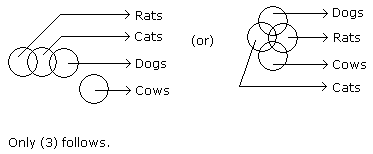Test: Syllogism- 3 - Question 30

Directions to Solve

In each of the following questions there are three statements. Which are followed by three or four conclusions. Choose the conclusions which logically follow from the given statements.

Question -

Statements: All the books are papers. Some papers are journals. Some journals are calendars.

Conclusions:

1. Some journals are books.
2. Some calendars are papers.
3. Some books are journals.
4. Some books are calendars.
Detailed Solution for Test: Syllogism- 3 - Question 30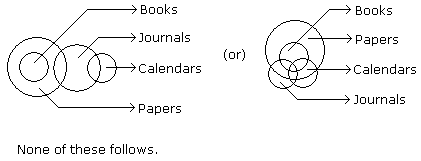## CSAT Preparation for UPSC CSE

72 videos|64 docs|92 tests
 Use Code STAYHOME200 and get INR 200 additional OFF Use Coupon Code
Information about Test: Syllogism- 3 Page
In this test you can find the Exam questions for Test: Syllogism- 3 solved & explained in the simplest way possible. Besides giving Questions and answers for Test: Syllogism- 3, EduRev gives you an ample number of Online tests for practice

## CSAT Preparation for UPSC CSE

72 videos|64 docs|92 tests